Note: This is an archvied version of our old webpage. Some links might be broken. The current one can be found here.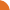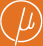Chair for Foundations of Software Reliability and Theoretical Computer Science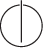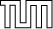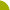Publications - Zero-reachability in probabilistic multi-counter automataReference:

Tomáš Brázdil, Stefan Kiefer, Antonín Kučera, Petr Novotný, and Joost-Pieter Katoen. Zero-reachability in probabilistic multi-counter automata. In Proceedings of the Joint Meeting of the Twenty-Third EACSL Annual Conference on Computer Science Logic (CSL) and the Twenty-Ninth Annual ACM/IEEE Symposium on Logic in Computer Science (LICS), pages 22:1–22:10, Vienna, Austria, 2014.

Abstract:

We study the qualitative and quantitative zero-reachability problem in probabilistic multi-counter systems. We identify the undecidable variants of the problems, and then we concentrate on the remaining two cases. In the first case, when we are interested in the probability of all runs that visit zero in some counter, we show that the qualitative zero-reachability is decidable in time which is polynomial in the size of a given pMC and doubly exponential in the number of counters. Further, we show that the probability of all zero-reaching runs can be effectively approximated up to an arbitrarily small given error epsilon > 0 in time which is polynomial in log(epsilon), exponential in the size of a given pMC, and doubly exponential in the number of counters. In the second case, we are interested in the probability of all runs that visit zero in some counter different from the last counter. Here we show that the qualitative zero-reachability is decidable and SquareRootSum-hard, and the probability of all zero-reaching runs can be effectively approximated up to an arbitrarily small given error epsilon > 0 (these results apply to pMC satisfying a suitable technical condition that can be verified in polynomial time). The proof techniques invented in the second case allow to construct counterexamples for some classical results about ergodicity in stochastic Petri nets.

Suggested BibTeX entry:

@inproceedings{14BKKNK-LICS,PDF (368 kB)Tech report version